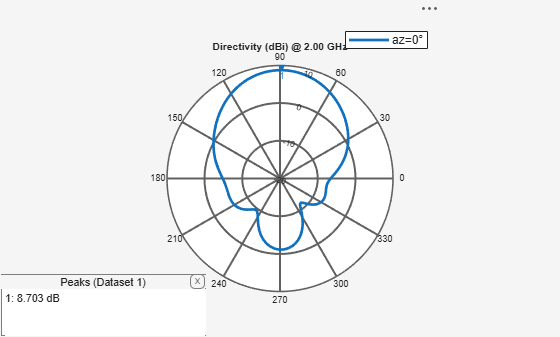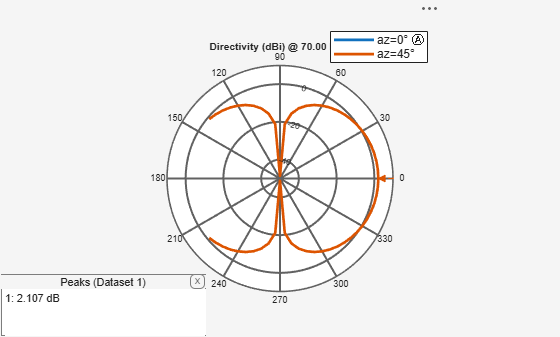Documentation

# patternElevation

Elevation pattern of antenna or array

## Syntax

``patternElevation(object,frequency,azimuth)``
``patternElevation(object,frequency,azimuth,Name,Value)``
``directivity = patternElevation(object,frequency,azimuth)``
``directivity = patternElevation(object,frequency,azimuth,'Elevation')``

## Description

example

````patternElevation(object,frequency,azimuth)` plots the 2-D radiation pattern of the antenna or array object over a specified frequency. Azimuth values defaults to zero if not specified.```

example

````patternElevation(object,frequency,azimuth,Name,Value)` uses additional options specified by one or more `Name, Value` pair arguments.```
````directivity = patternElevation(object,frequency,azimuth)` returns the directivity of the antenna or array object at specified frequency. Azimuth values defaults to zero if not specified.```
````directivity = patternElevation(object,frequency,azimuth,'Elevation')` uses additional options specified by one or more `Name, Value` pair arguments.```

## Examples

collapse all

Calculate and plot the elevation pattern of the helix antenna at 2 GHz.

```h = helix; patternElevation (h, 2e9);```Calculate and plot the elevation radiation pattern of the dipole antenna at 70 MHz at elevation values of 0 and 45.

``` d = dipole; patternElevation(d,70e6,[0 45],'Elevation',-140:5:140);```## Input Arguments

collapse all

Antenna or array object, specified as a scalar handle.

Frequency used to calculate charge distribution, specified as a scalar in Hz.

Example: 70e6

Data Types: `double`

Azimuth angle values, specified as a vector in degrees.

Example: `[0 45]`

Data Types: `double`

Elevation angles of antenna, specified the comma-separated pair consisting of `'Elevation'` and a vector in degrees.

Example: `'Elevation'`, 0:1:360

Data Types: `double`

## Output Arguments

collapse all

Antenna or array directivity, returned as a matrix in `dBi`. The matrix size is the product of number of elevation values and number of azimuth values.

## See Also

#### Hybrid Beamforming for Massive MIMO Phased Array Systems

Download the white paper